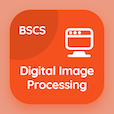Computer Science Courses Online

Digital Image Processing Prep Tests

Digital Image Processing Tests

The Book Background of Intensity Transformation Multiple Choice Questions (MCQ Quiz), Background of Intensity Transformation quiz answers PDF to learn online courses, digital image processing tests. Study Intensity Transformation and Spatial Filtering Multiple Choice Questions and Answers (MCQs), Background of Intensity Transformation quiz questions for best online schools for computer science. The eBook Background of Intensity Transformation MCQ App Download: image negatives, power law transformation, smoothing spatial filters, histogram equalization test prep for accelerated computer science degree online.

The MCQ: In the formula g(x,y) = T[ƒ(x,y)], T is the PDF, "Background of Intensity Transformation" App Download (Free) with transformed image, transformation vector, transformation theorem, and transformation function choices for best online schools for computer science. Practice background of intensity transformation quiz questions, download Amazon eBook (Free Sample) for online software development courses.

## Image Processing: Background of Intensity Transformation MCQs

MCQ: In the formula g(x,y) = T[ƒ(x,y)], T is the

A) transformed image
B) transformation vector
C) transformation theorem
D) transformation function

MCQ: Fourier stated that the periodic function is expressed as sum of

A) sine
B) cosine
C) tangent
D) Both A and B

A) smoothing
B) sharpening
D) Both A and B

MCQ: In the formula g(x,y) = T[ƒ(x,y)], ƒ(x,y) is the

A) transformed image
B) output image
C) input image
D) digitized image

MCQ: The Fourier transform is named after French mathematician

A) joseph Fourier
B) john Fourier
C) sean Fourier
D) jay Fourier

### Practice Tests: Digital Image Processing Exam Prep

Download Digital Image Processing Quiz App, Computer Networks MCQs App and DBMS MCQ App for Android & iOS devices. These Apps include complete analytics of real time attempts with interactive assessments. Download Play Store & App Store Apps & Enjoy 100% functionality with subscriptions!